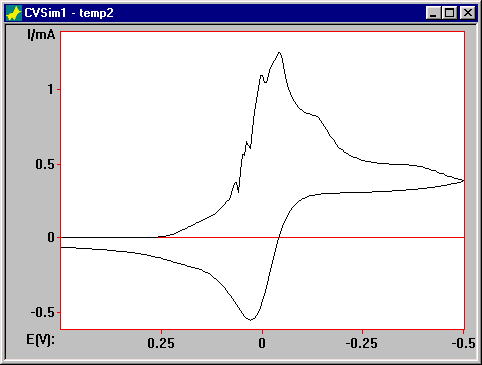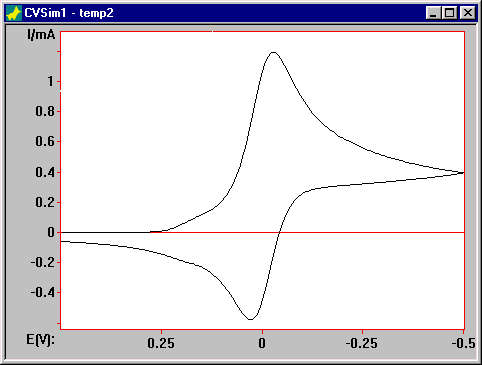# Digisim® Tutorials

#### 5. How Do You Know It's Right?

The results obtained using any numerical computation should always be examined critically to ensure that the answer is reasonable, and DigiSim® is no exception to this rule. One approach that can be used is to compare simulated voltammograms with analogous voltammograms from the literature. However, there is no guarantee that mechanisms proposed in the literature are correct (e.g., some reactions may have been omitted). Another approach that is generally more useful for DigiSim is to vary the computational parameters.

The exponential time and space grids used by the implicit finite difference computation are characterized by Dt and Dx. Although these parameters are not defined explicitly in the user interface, their values can be altered by changing the Potential steps and the Expanding grid factor, respectively, in the Model Parameters dialog box. Decreasing the values of these parameters almost always improves the accuracy of a given simulation, but the computation time is also increased. DigiSim sets default values for these parameters that will produce acceptable accuracy (better than 0.5%) in most cases. However, there are instances where the particular set of parameter values used causes computational problems, which can be eliminated by decreasing the values of one or both of these Model Parameters.

This can be illustrated by the catalytic reaction:

 A + e = B (Eo = 0 V, [A] = [C] = 0.001 M) B + C = A + D (Keq = 1000, kf = 1e10, DC = DD = 1e-7)

(leave any unspecified parameters at the default value).

There are two points of note:

a) the rates of the homogeneous reactions are very fast
b) the diffusion coefficients of C and D are two orders of magnitude smaller than those of A and B

The simulation obtained using the default values of the Expanding grid factor and the Potential Steps (0.5 and 0.005 V, respectively) obviously cannot be correct (F1).Figure 1. Simulation of for the catalytic mechanism using the default Model Parameter values.

If the Expanding grid factor is changed to 0.05, there is a considerable improvement in the simulation (F2).Figure 2. Simulation of for the catalytic mechanism with the Expanding grid factor = 0.05.

Further decreases in the value of of the Expanding grid factor produce no further improvement, which is a good indication that the voltammogram in F2 is accurate.

In the above example, it was obvious that the voltammogram for the default Model Parameters was incorrect. However, inaccuracies in voltammogram are generally not as obvious - it is possible to obtain a simulated voltammogram that looks reasonable but is still inaccurate. It is good practice to run any simulation using different values for the Expanding grid factor and the Potential Steps to check for accuracy.

Another Model Parameter that can affect the accuracy of the simulation is the number of Gauss-Newton iterations. The Gauss-Newton (GN) method is used for solving both linear and non-linear equations. When the equations are linear (which arise for one-electron transfer reactions and first-order homogeneous reactions), the GN method only requires one iteration to obtain the exact answer. However, for non-linear equations (which arise for second-order homogeneous reactions), more than one iteration may be required in order to obtain an accurate result. Therefore, changing the value of this parameter to 2 or more can significantly affect the accuracy of the simulation.

DigiSim is a registered trademark of Bioanalytical Systems, Inc.

Copyright 1995-2000, BASi® E'CHEM Electrochemical Products Group

Back to Tutorials Index